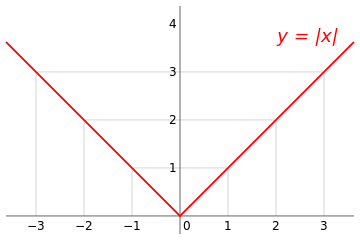# What is f(x)?

Algebra Level 4Let $f\colon\mathbb{R}\to\mathbb{R}$ be a function that satisfies the following property.

For all $x\in \mathbb{R}$, $f(x)^2=x^2$.

Consider the following statements.

$$. $f(x)$ has to be equal to $x$ for all $x\in\mathbb{R}$.

$$. $f(x)$ has to be equal to $-x$ for all $x\in\mathbb{R}$.

$$. In fact, $f(x)$ could be one of three things. $f(x)=x$ for all real $x$, $f(x)=-x$ for all real $x$ and $f(x)=|x|$ for all real $x$

$$. It is impossible to tell what $f(x)$ is.

Which of these statements is correct?

×

Problem Loading...

Note Loading...

Set Loading...